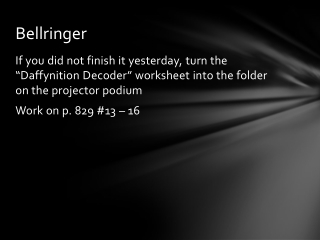DownloadDownload PresentationBellringer

# Bellringer

Télécharger la présentation## Bellringer

- - - - - - - - - - - - - - - - - - - - - - - - - - - E N D - - - - - - - - - - - - - - - - - - - - - - - - - - -
##### Presentation Transcript

1. Bellringer • If you did not finish it yesterday, turn the “Daffynition Decoder” worksheet into the folder on the projector podium • Work on p. 829 #13 – 16

2. Section 8-1: Pythagorean Theorem and its Converse SPI 3108.4.14 Use properties of right triangles to solve problems

3. Pythagorean Theorem • If a triangle is a right triangle, then the sum of the squares of the lengths of the legs is equal to the square of the length of the hypotenuse • a2 + b2 = c2 • Always make sure to check which side is the hypotenuse. That side goes in for c.

4. ACT Example • If a rectangle measures 54 meters by 72 meters, what is the length, in meters, of the diagonal of the rectangle? • A. 48 • B. 63 • C. 90 • D. 126 • E. 252

5. Simplest Radical Form • Many problems will say, “Express your answer in simplest radical form” • Steps: • Break your answer up by using a factor tree • Look for and circle pairs at the bottom of tree • Numbers in the circles come out, numbers not in circles stay inside • If more than one number comes in or stays out, then multiply

6. Converse of Pythagorean Theorem • If a2 + b2 = c2 , then the triangle is a right triangle • If a2 + b2> c2, then the triangle is acute • If a2 + b2< c2, then the triangle is obtuse

7. Homework • Pythagorean Theorem Worksheet • Quiz on Tuesday